The MCAT CARS Strategy Course begins January 28!

MCAT Content / Electronic Structure / Conventional Notation For Electronic Structure

### Conventional notation for electronic structure

Topic: Electronic Structure

The arrangement of electrons in the orbitals of an atom is called the electron configuration of the atom.

We describe an electron configuration with a symbol that contains three pieces of information:

– The number of the principal quantum shell. For the example below: n =1
– The letter designates the orbital type s p d or f (the subshell of angular momentum).
– A superscript number that designates the number of electrons in that particular subshell.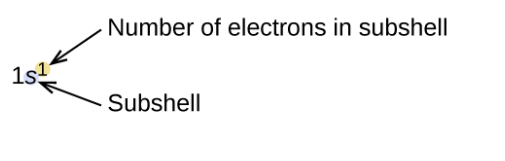As the number of electrons in the atoms increased, each added electron occupies the subshell of lowest energy available, with 2 electrons per orbitals. Electrons enter higher-energy subshells only after lower-energy subshells have been filled.The Aufbau principle, as shown in the figure above, illustrates the traditional way to remember the filling order for atomic orbitals. For example, the 5p orbitals fill immediately after the 4d, and immediately before the 6s.

Orbital diagrams are pictorial representations of the electron configuration, showing the individual orbitals and the pairing arrangement of electrons. We start with a single hydrogen atom (atomic number 1), which consists of one proton and one electron.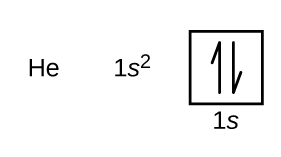The n = 1 shell is filled in a helium atom, and the electrons are spin paired.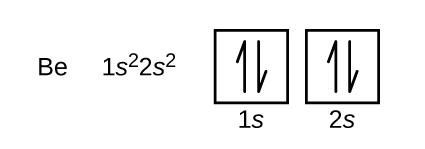The fourth electron of beryllium fills the remaining space in the 2s orbital.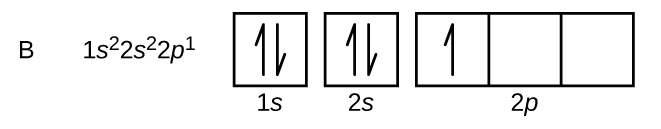An atom of boron (atomic number 5) contains five electrons. The n = 1 shell is filled with two electrons, and three electrons will occupy the n = 2 shell. Because any s subshell can contain only two electrons, the fifth electron must occupy the next energy level, which will be a 2p orbital. There are three degenerate 2p orbitals (ml = −1, 0, +1), and the electron can occupy any one of these p orbitals. When drawing orbital diagrams, we include empty boxes to depict any unoccupied orbitals in the same subshell that we are filling.Carbon (atomic number 6) has six electrons. Four of them fill the 1s and 2s orbitals. The remaining two electrons occupy the 2p subshell. The orbitals are filled as described by Hund’s rule: having the maximum number of unpaired electrons for the lowest-energy configuration.

The orbital diagram of the following elements can be filled as below.A core-abbreviated electron configuration (right) replaces the core electrons with the noble gas symbol whose configuration matches the core electron configuration of the other element.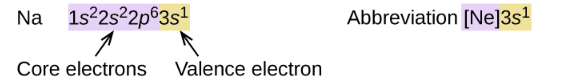Practice Questions

MCAT Official Prep (AAMC)

Chemistry Online Flashcards Question 3

Chemistry Question Pack Question 73

Chemistry Question Pack Passage 14 Question 79

Chemistry Question Pack Passage 19 Question 106

Section Bank C/P Section Question 25

Practice Exam 3 C/P Section Passage 3 Question 15

Key Points

• The relative energy of the subshells determines the order in which atomic orbitals are filled (1s, 2s, 2p, 3s, 3p, 4s, 3d, 4p, and so on).

• Electron configurations and orbital diagrams can be determined by applying the Pauli exclusion principle (no two electrons can have the same set of four quantum numbers) and Hund’s rule (whenever possible, electrons retain unpaired spins in degenerate orbitals).

Key Terms

Atom: the smallest possible amount of matter that still retains its identity as a chemical element. Atoms consist of a nucleus surrounded by electrons.

Orbital: physical region or space where the electron can be calculated to be present.

Orbital diagrams: pictorial representations of the electron configuration.

Aufbau principle: states that in the ground state of an atom or ion, electrons fill atomic orbitals of the lowest available energy levels before occupying higher levels.

Degenerate: mean ‘of equal energy.’

Hund’s rule: every orbital in a subshell is singly occupied with one electron before any one orbital is doubly occupied

Billing Information